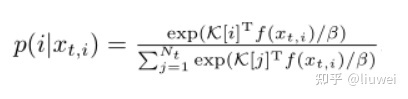backbone：

neck:

bottleneck:

GAP：

`self.gap = nn.AdaptiveAvgPool2d(1)`

Embedding:

temperature parameters```import torch
x = torch.tensor([1.0,2.0,3.0])
y = torch.softmax(x,0)
print(y)

x1 = x / 2  # beta 为2
y = torch.softmax(x1,0)
print(y)

x2 = x/0.5  # beta 为0.5
y = torch.softmax(x2,0)
print(y)```

```tensor([0.0900, 0.2447, 0.6652])
tensor([0.1863, 0.3072, 0.5065])
tensor([0.0159, 0.1173, 0.8668])```

Warm up指的是用一个小的学习率先训练几个epoch，这是因为网络的参数是随机初始化的，一开始就采用较大的学习率容易数值不稳定。

end to end